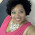## Monday, 7 October 2013

### Bartlett's Test: Equality of variances

There are statistical tests that involve two or more datasets, and that rely on the assumption that all the datasets have the same variance. For example, the commonly used version of the Unpaired Student's t-test assumes that the two datasets have the same variance.

Another widely used test that assumes that three or more datasets have the same variance is the one-way and two-way ANOVA test.

Bartlett's test can be used to determine whether the datasets (or groups) have the same variance, and relies on the assumption that all the datasets come from a Normal distribution.

Suppose we have $k$ groups/datasets.

We first calculate the variance for each of the $k$ groups as follows ($i=1,2,..,k$):
$\Large \sigma_i^2=\frac{1}{N_i-1}\sum_{n=1}^{N_i}(x_i[n]-\mu_i)^2$
where $N_i$ is the number of samples in the $ith$ group, and $\mu_i$ is the mean of the $ith$ group.
$\Large \mu_i=\frac{1}{N_i}\sum_{n=1}^{N_i}x_i[n]$

Next we calculate the pooled variance $\sigma_p^2$
$\Large \sigma_p^2=\frac{1}{N-k}(\sum_{i=1}^k(N_i-1)\sigma_i^2)$
where $N$ is the total number of samples for all $k$ groups, i.e.
$N=\sum_{i=1}^kN_i$

Once we have the variances for the $k$ groups and the pooled variance, we are in a position to calculate the Bartlett statistic $X^2$, which is given by

$\Large X^2=\frac{(N-k)ln(\sigma_p^2)-\sum_{i=1}^k(N_i-1)ln(\sigma_i^2)}{1+\frac{1}{3(k-1)}(\sum_{i=1}^k(\frac{1}{N_i-1})-\frac{1}{N-k})}$

Now the statistic $X^2$ (approximately) has a chi-squared distribution $\chi_{k-1}^2$.

Assuming that the Null Hypothesis is that the variances for all $k$ groups are the same, we reject this (i.e. the test result is significant, hence the assumption that the variances are all the same is rejected) if the calculated $X^2$ exceeds $\chi_{(k-1),\alpha}^2$, the critical value of the chi-squared distribution.

This will be the case when $X^2$ falls within the right tail region of the chi-squared distribution.

The parameter $\alpha$ is 1 minus the significance level. For a 5% significance level, $\alpha=1-0.05=0.95$ - note that this is a one-tailed test, as the variance is always greater than zero.

To find the value $\chi_{(k-1),\alpha}^2$, you need to calculate the inverse CDF of the chi-squared distribution for given $\alpha$ and $k$. This can be done using various statistical packages, or using tables, or (yes you guessed correctly..) using my iOS App SciStatCalc.

#### 1 comment:

1.Good luck to anyone reading this true life story of mine, I Was Diagnosed With type 2 Herpes Virus Last year, And I Was Looking For Solution To Be Cured Luckily I Saw Testimonies On How Dr OYAGU Cure Herpes Virus I Decided To Contact Dr OYAGU I Contacted Him He Prepared A Herbal Medicine Portion And Sent It To Me, I Started The Herbal Medicine For My Health. He Gave Me Step By Step Instructions On How To Apply It, When I Applied It As Instructed, I Was Cured Of This Deadly Herpes Within 2 weeks, I Am Now Herpes Negative. My Brother And Sister I No That There Are So Many People That Have The Same Herpes Virus Please contact Dr OYAGU To Help You Too, And Help Me To Thank Dr OYAGU For Cure Me, I’m Cured By Dr. OYAGU Herbal Medicine, His Contact Email:oyaguherbalhome@gmail.com or visit his website https://oyaguspellcaster.wixsite.com/oyaguherbalhome Or Cell Whatsapp Number +2348101755322 thank you